Home » Getting your clustering right (Part I)

# Getting your clustering right (Part I)

Clustering is one of the toughest modelling techniques.

It takes not only sound technical knowledge, but also good understanding of business. We have split this topic into two articles because of the complexity of the topic. As the technique is very subjective in nature, getting the basics right is very critical.

This article will take you through the basics of clustering. The next article will get into finer details of the technique and identify certain scenarios where the technique fails. The article will also introduce to a simple method to counter such scenarios.

What is clustering analysis?

Clustering analysis is the task of grouping a set of objects in such a way that objects in the same group (called a cluster) are more similar (in some sense or another) to each other than to those in other groups (clusters). Following figure is an example of finding clusters of US population based on their income and debt :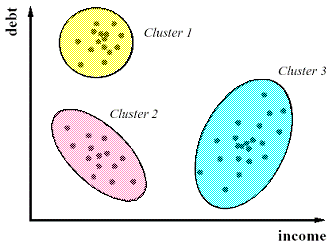It is one of the subjective modelling technique widely used in the industry. One of the examples of common Clustering usage is segmenting customer portfolio based on demographics, transaction behavior or other behavioral attributes.

Why do we need clustering ?

Analytics industry is dominated by objective modelling like decision tree and regression. If decision tree is capable of doing segmentation, do we even need such an open ended technique? The answer to this question is in one of the advantages of using clustering technique. Clustering generates natural clusters and is not dependent on any driving objective function. Hence such a cluster can be used to analyze the portfolio on different target attributes. For instance, say a decision tree is built on customer profitability in next 3 months. This segmentation cannot be used for making retention strategy for each segment. If segmentation were developed through clustering, both retention and profitability strategy can be built on these segments.

Hence, clustering is a technique generally used to do initial profiling of the portfolio. After having a good understanding of the portfolio, an objective modelling technique is used to build specific strategy.

Industry standard techniques for clustering :

There are a number of algorithm for generating clusters in statistics. But we will discuss in detail only two such techniques which are widely used in the industry. These techniques are as follows :

1. Hierarchical Clustering : This technique operate on the simplest principle, which is data-point closer to base point will behave more similar compared to a data-point which is far from base point. For instance, a , b ,c, d, e,f are 6 students, and we wish to group them into clusters.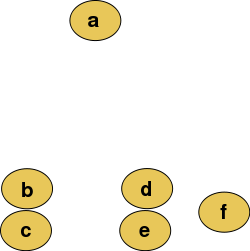Hierarchical Clustering will sequentially group these students and we can stop the process at any number of clusters we want. Following is an illustrative chain of clustering :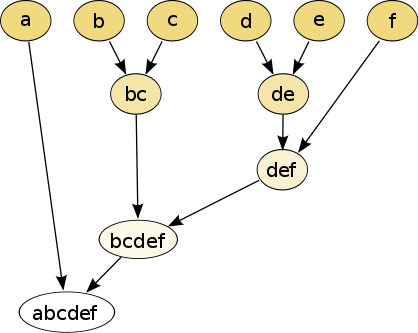Hence, if we want 3 clusters, a , bc and def are the required clusters. So far so simple. The technique uses the very basic of clustering and is, therefore, a very stable technique.

The only problem with the technique is that it is able to only handle small number of data-points and is very time consuming. This is because it tries to calculate the distance between all possible combination and then takes one decision to combine two groups/individual data-point.

2. k-means Clustering : This technique is more frequently used in analytics industry as it is able to handle large number of data points. FASTCLUS is an algorithm used by SAS to generate k-means cluster. Lets try to analyze how it works.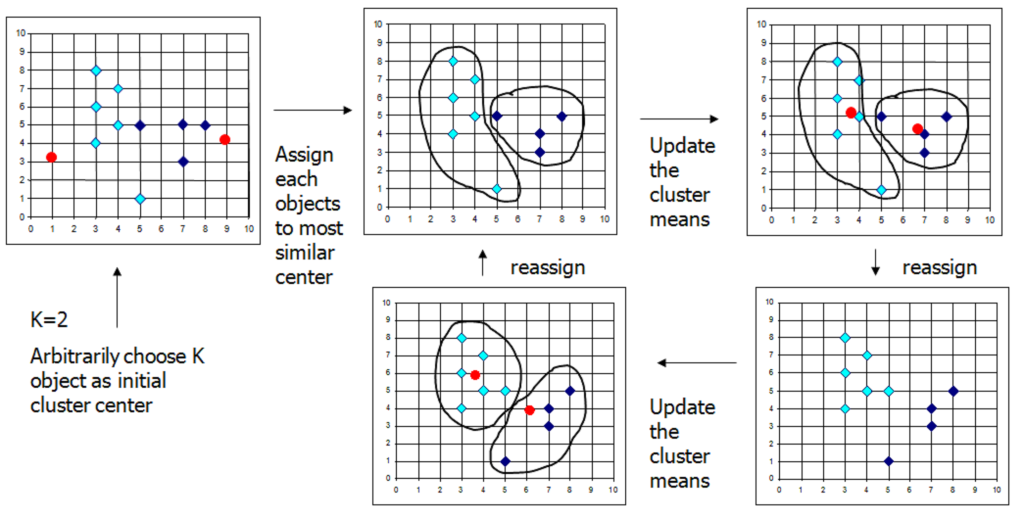As can be seen from the figure above, we start with a definite number for the number of required cluster (in this case k=2). The algorithm takes 2 random seeds and maps all other data points to these two seeds. The algorithm re-iterates till the overall penalty term is minimized.

When we compare the two techniques, we find that the Hierarchical Clustering starts with individual data-points and sequentially club them to find the final cluster whereas  k-means Clustering  starts from some initial cluster and then tries to reassign data-points to k clusters to minimize the total penalty term. Hence for large number of data-points,   k-means uses far lesser iterations then Hierarchical Clustering.

Steps to perform cluster analysis:

Having discussed what is clustering and its types, lets apply these concepts on a business case. Following is a simple case we will try to solve :

US bank X wants to understand the profile of its customer base to build targeted campaigns.

Step 1 – Hypothesis building : This is the most crucial step of the whole exercise. Try to identify all possible variables that can help segment the portfolio regardless of its availability. Lets try to come up with a list for this example.

a. Customer balance with bank X

b. Number of transaction done in last 1/3/6/12 months

c. Balance change in last 1/3/6/12 months

d. Demographics of the customer

e. Customer total balance with all US banks

The list is just for illustrative purpose. In real scenario this list will be much longer.

Step 2 – Initial shortlist of variable : Once we have all possible variable, start selecting variable as per the data availability. Lets say, for the current example we have only data for Customer balance with bank X and Customer total balance with all US banks (total balance)

Step 3 – Visualize the data : It is very important to know the population spread across the selected variable before starting any analysis. For the current scenario, the exercise becomes simpler as the number of selected variables is only 2. Following is a scatter plot between total balance and Bank X balance (origin taken as mean of both the variables):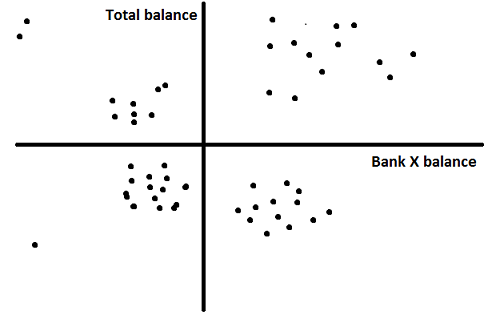This visualization helps me to identify clusters which I can expect after the final analysis. Here, we can see there are four clear clusters in four quadrants. We can expect the same result in the final solution.

Step 4 – Data cleaning : Cluster analysis is very sensitive to outliers. It is very important to clean data on all variables taken into consideration. There are two industry standard ways to do this exercise :

1. Remove the outliers : (Not recommended in case the total data-points are low in number) We remove the data-points beyond mean +/- 3*standard deviation.

2. Capping and flouring of variables : (Recommended approach) We cap and flour all data-points at 1 and 99 percentile.

Lets use the second approach for this case.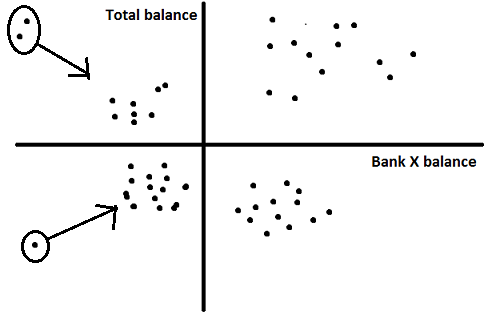Step 4 – Variable clustering :  This step is performed to cluster variables capturing similar attributes in data. And choosing only one variable from each variable cluster will not drop the sepration drastically compared to considering all variables. Remember, the idea is to take minimum number of variables to justify the seperation to make the analysis easier and less time consuming. You can simply use Proc VARCLUS to generate these clusters.

Step 5 – Clustering : We can use any of the two technique discussed in the article depending on the number of observation. k-means is used for a bigger samples. Run a proc fastclus with k=4 (which is apparent from the visualization).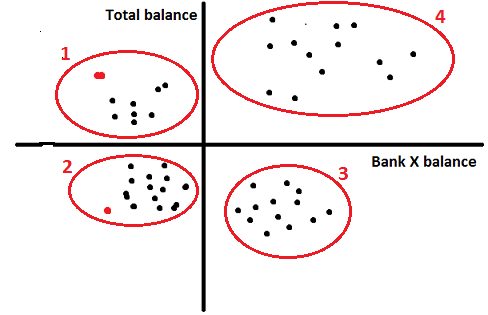As we can see, the algorithm found 4 clusters which were already apparent in the visualization. In most business cases the number of variables will be much larger and such visualization won’t be possible and hence

Step 6 – Convergence of clusters : A good cluster analysis has all clusters with population between 5-30% of the overall base. Say, my total number of customer for bank X is 10000. The minimum and maximum size of any cluster should be 500 and 3000. If any of the cluster is beyond the limit than repeat the procedure with additional number of variables. We will discuss in detail about other convergence criterion in the next article.

Step 7 – Profiling of the clusters : After validating the convergence of cluster analysis, we need to identify behavior of each cluster. Lets say we map age and income to each of the four clusters and get following results :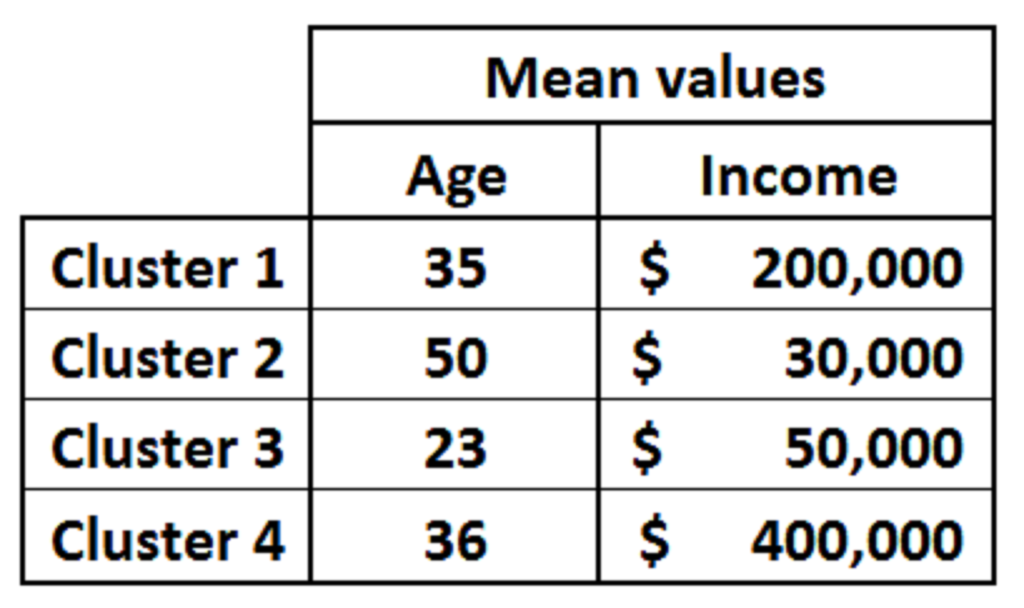Now is the time to build story around each cluster. Lets take any two cluster and analyze.

Cluster 1 : (High Potential Low balance customer) These customers do have high balance in aggregate but low balance with bank X. Hence, they are high potential customer with low current balance. Also the average salary is on a higher side which validates our hypothesis of customer being high potential.

Cluster 3 : (High Potential high balance customers) Even though the salary and total balance in aggregate is on a lower side, we see a lower average age. This indicates that the customer has a high potential to increase their balance with bank X.

Final notes :

As we saw, using clusters we can understand the portfolio in a better way. We can also build targeted strategy using the profiles of each cluster. In the Part 2 of this article we will discuss following :

1. When is cluster analysis said to be conclusive?

2. Different scenarios in which each of the two techniques dominate?

3. When do both techniques fail?

4. Step by step solution in a scenario when both the techniques fail.

When do you use unsupervised modelling techniques? Do you use any other method for clustering often? What challenges do you face while building clusters? Do let us know your thoughts in comments below.

## If you like what you just read & want to continue your analytics learning, subscribe to our emails or like our facebook page.

You can also read this article on our Mobile APP•igor says:

Hi Tavish,

what is your recommendation for visualizing the data for more than 3 variables?

Also, which distance/ similarity functions are most commonly used for K means clustering ?
Is there a more preferable distance/ similarity function for specific areas/problems ?

Thanks.

•Tavish says:

Igor,
1. Visualization : Visualization of data spread is easy till 3 variables. Beyond 3 variable there are two approaches :

a. Distribution analysis : Check the univariate and bivariate plots for all variable combinations. In case you have one or two very significant variables, our job becomes easy. Take a univariate and bivariate only on those variables and you will find a good seperation between clusters.Provided outlier treatment is thoroughly done, univariate plaots give a good indication.

b. Visual technique : Take 3 most significant variable as basic dimensions and make bins of rest of the variables. Now plot a 3-D curve for each bin.This technique works well for 4 variables but becomes difficult in larger number of variables.

2. K-means operates on the objective : “find the cluster centers and assign the objects to the nearest cluster center, such that the squared distances from the cluster are minimized.”

3. Minimize squared distance technique is most widely used technique across industry. For a specific scenario i.e. the population is very uniform, please read the next part of this article http://www.analyticsvidhya.com/blog/2013/11/getting-clustering-right-part-ii/

We have not discussed the parameters we check to find the best technique which fits in the problem in hand because of the mathematical details we will need to cover. However, if you have any doubts on these parameter, feel free to ask.

Hope this helps.
Tavish

•igor says:

Hi Tavish,

How you can actually determined which of the variables are the most significant ?
Is there any statistical test that can help in such case (especially when you have >7 variables) ?
Also, I would like to ask, Is cluster analysis can be done for categorical variables (will the minimize squared distance technique fits in such case as well) ? A mix of categorical variables with covariate variables ?

Thanks,
Igor

•Tavish says:

Hi Igor,
1. In cases when you have more than 10 variables, we normally do variable shortlisting in two steps. The first step is by finding variable clusters. As the name suggests, this step makes clusters of all variables. Now based on the least (1-Rsquare) function, choose 1 or 2 variables from each cluster. The second step is by creating observation cluster by using FASTCLUS. You will get the value for Rsquare/(1-Rsquare) for each variables. The one with lowest value can be dropped without changing cluster definitions significantly.
2. Yes you can use categorical variables alone or with continous variables to build clusters. Cluster definition is based on minimized distance on vector of each observation and hence can take only categorical variables as well. But prefer taking continous variables over categorical variables. Till date, I have not used any covariate variable in the model. But I can think of a method to do this as well. We can take a directional objective variable and build a decision tree. And create co-variate terms using this tree and add these created variables in your cluster analysis. To know how to make covariate terms, you can check out link : http://www.analyticsvidhya.com/blog/2013/10/trick-enhance-power-regression-model-2/

Hope this helps.
Tavish

•igor says:

What do you thing about using PCA for each cluster in order to determine the most significant variables
in each cluster ?

Thanks,

•Tavish Srivastava says:

Igor,
I have not used Principal component procedure for cluster analysis, because of the simplicity of using VARCLUS and FASTCLUS in conjuction for cluster analysis iterations (you will make many iterations to get to good clusters) . But, I dont see any challenge using PCA technique. Please do share your experience if you use PCA in your cluster analysis.

Tavish

•Anuj says:

You have mentioned the terms Objective and Subjective modelling, can you please give a brief introduction of these techniques?

Also what is meaning of the ‘penalty term’ in case of K means clustering ? what actually the seed is, is it a random point from where we are measuring distance of all the point?

Thanks

•Tavish Srivastava says:

Hi Anuj,
1. Objective modelling are techniques with an objective/target variable. Say, I want to find segment of customer with high profitibilty. Here profit generated by the customer becomes the objective or target variable. Some of the objective modelling techniques are CART,CHAID, Linear regression and logistic regression.Subjective modelling are techniques without a target variable. Here we try to find natural clusters or groups. Observations similar are clubbed together and different are kept in different clusters. Clustering is one of the subjective modelling techniques.
2. Penalty term of k means is the sum of distance of observations to the cluster seed. When this penalty term is minimum, it implies that similar observations have been clubbed and different observations have been seperated. K means algorithm starts with alloting seed randomly to k number of observations. The algorithm now assigns different observation to one of the seeds. Once this assignment is done, centroid of each cluster is designated as seed. The reassignment of observation to seeds again takes place. This process repeates till the penalty term is minimized.
Do let me know in case you still have doubts.
Tavish

•Anuj says:

Just a small question, as per my limited knowledge there should always be an objective attached to whatever is performed. I mean in case of subjective modelling if we are forming clustering, (which I understand is a kind of segregation of similar type of data values) must be base to something, like may be base to Objective modelling which may be performed on top of this.

Thanks

•Tavish Srivastava says:

Anuj,
You are right, all modelling techniques have an objective function attached to it.But here I am referring to objective or target variable and not objective function.

Let me try to rephrase the difference between objective and subjective techniques. Objective modelling involves one and only one target function. For instance, I have a pool of customers and I wish to find the segment this customer base to do targeted engagement with each segment of customers. Lets try to solve this problem using both the techniques.

1. Subjective modelling : We find similar customers based on their buying patterns, demographics etc. There was no objective or target function but still have to minimize an objective function i.e. sum of distances of observation from their corresponding seeds.
2. Objective modelling : We take attrition rate as the objective/target function, and build a CART. Now, I have segments most likely to attrite. We can build corresponding retention strategy for segments with high attrition rate. Here, the objective was to maximize the difference between attrition rates of the segments and the objective / target function was attrition rate.
As you can see, the objective modelling had a target variable which gives direction to the model but subjective model has no such variable. Few observations on this example are :
1. Objective models are more robust and replicable. Subjective models is highly dependent on the assumptions taken by the modeler.
2. Subjective models are not directional and hence can be used for multiple purposes. For this example, objective model can be used for building retention strategy only, but subjective model can be used for retention strategy, X-sell, up-sell etc. depending on the profiles of clusters.

I hope this clarifies your doubt.

Tavis

•Anuj says:

Hi Tavish,

Thanks a lot for the explanation, it is very much clear now. But here one small question again, like Clustering is one of the techniques that we use for Subjective modelling, similarly there must be techniques/procedures for Objective modelling also. Can you please name some of those.

Thanks

•Tavish says:

Anuj,
Some of the objective modelling techniques are CART,CHAID, linear regression,logistic regression etc.
Tavish

•igor says:

Hi Tavish,

I may miss understood something. Suppose the categorical variable can have 3 diff values
“Intern”, “Junior”, “Senior” , how can you at all measure the distance between the 3 different values ?
Is “Junior’ closer to “Intern” or to “Senior” ?

Thanks.

•Tavish says:

Igor,

We use dummy variables to tackle such categorical variables. For your example, we will create two variables i.e. Int and Jun. Following will be the values of the 2 variables created for the three levels :

Intern Int(1) Jun(0)
Junior Int(0) Jun(1)
Senior Int(0) Jun(0)

Now by including Int and Jun in the model you will be able to capture all the three levels. Do let me know in case you have any further doubts.

Tavish

•Tiago says:

Would you mind if I use the first figure for my thesis report?

•Aman says:

Hi Tavish,

According to my limited experience, one of the most important aspect in Unsupervised clustering using K-means is deciding the number of clusters to be created.

One of the methods suggested by you is Visualizing the cluster using scatter plot but as you told that in dataset having large number of variables, this might work.

So, we can also use “Elbow curve” to determine number of clusters.
here the objective will be to minimize intra-cluster distance and maximize inter-cluster distance i.e. getting very distinct clusters.

Also, for dimension reduction we might also use principle component analysis.

•Eric says:

Great article – what are other “subjective modeling” techniques besides cluster analysis?

•Chame says:

How do we do segment the customers in a survey response data where customer responses are 5 scaled. One way is to group the customers in 5 groups on the ratings they have given, but can we use cluster analysis to group the customers in a different manner?

•Ravi says:

Hi Tavish,

Thanks for this wonderful article. I have a query though, would really appreciate if you or any AVian could explain it.
In the example of the article, how do you decide on the variables to consider for clustering, you had Age & Income data available along with the ‘customer balances with X/All’ variables, but you haven’t included them for clustering, while they were considered in profiling of customers. Please help me understand the variable selection thought process for clustering & profiling of customer, and how do they differ.

Regards,
Ravikant